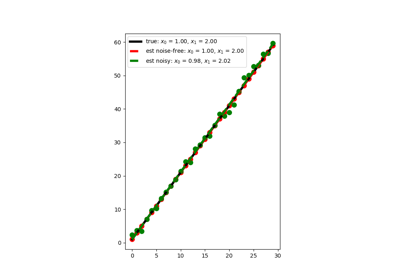# pylops.LinearRegression¶

pylops.LinearRegression(taxis, dtype='float64')[source]

Linear regression.

Creates an operator that applies linear regression to a set of points. Values along the $$t$$-axis must be provided while initializing the operator. Intercept and gradient form the model vector to be provided in forward mode, while the values of the regression line curve shall be provided in adjoint mode.

Parameters: taxis : numpy.ndarray Elements along the $$t$$-axis. dtype : str, optional Type of elements in input array. TypeError If taxis is not numpy.ndarray.

Regression
Polynomial regression

Notes

The LinearRegression operator solves the following problem:

$y_i = x_0 + x_1 t_i \qquad \forall i=0,1,\ldots,N-1$

We can express this problem in a matrix form

$\mathbf{y}= \mathbf{A} \mathbf{x}$

where

$\mathbf{y}= [y_0, y_1,\ldots,y_{N-1}]^T, \qquad \mathbf{x}= [x_0, x_1]^T$

and

$\begin{split}\mathbf{A} = \begin{bmatrix} 1 & t_{0} \\ 1 & t_{1} \\ \vdots & \vdots \\ 1 & t_{N-1} \end{bmatrix}\end{split}$

Note that this is a particular case of the pylops.Regression operator and it is in fact just a lazy call of that operator with order=1.

Attributes: shape : tuple Operator shape explicit : bool Operator contains a matrix that can be solved explicitly (True) or not (False)

## Examples using pylops.LinearRegression¶Linear Regression